STEMLabs IQ
Lab 12 - M.A.D. Box

Teacher Portal

# The M.A.D. Box's Gears

### Step 1: M.A.D. Box's Step 2: 12 and 36 Tooth Gears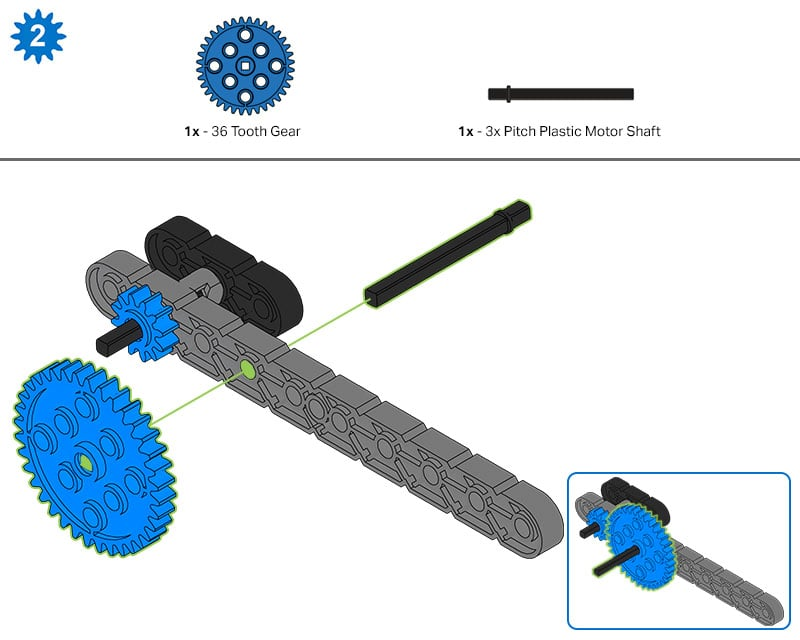In Step 2 of the Build Instructions, the 12 Tooth Gear was already on the shaft that connected the M.A.D. Box's handle on that side of the build.

• Build Expert, find that side of the M.A.D. Box and show it to your teammates. Then demonstrate that when that handle is turned, the shaft turns the 12 Tooth Gear (driving gear - input) which then turns the 36 Tooth Gear (driven gear - output) that is being added in this step of the build.

• What is the gear ratio of these two gears?

• Calculator, figure out the equation below and have the Recorder check it.Teacher Toolbox - Solution

The Calculator and Recorder in the group should recognize that the calculation should be completed as follows:

• The first fraction should be 36/12.

• The second fraction should be 3/1.

Again, ensure that students understand how to reduce fractions by diving both the numerator and denominator by the same number.

The 3:1 ratio tells us that the driving 12 Tooth Gear needs to turn three times in order to turn the 36 Tooth Gear once.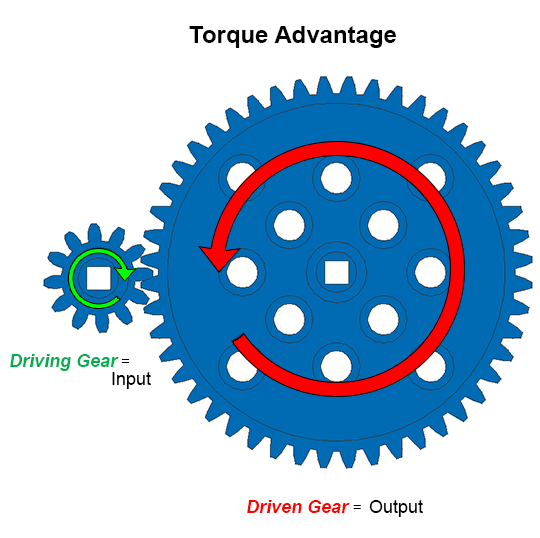Torque is a mechanical advantage that makes the output of the driven gear or machine more powerful. In this case, the M.A.D. Box had three times as much input as output which makes it more powerful.

• Recorder, be sure to add notes to the engineering notebook about the mechanical advantage of torque within the M.A.D. Box.

### Step 2: M.A.D. Box's Step 10: 36 and 12 Tooth Gears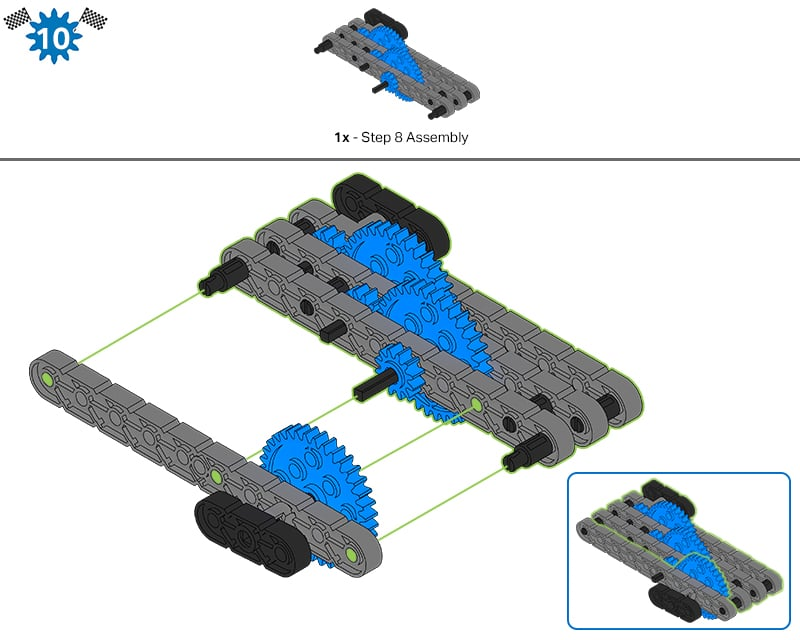In Step 10 of the Build Instructions, the other side of the M.A.D. Box was connected. It had a 36 Tooth Gear on the shaft with the handle.

• Build Expert, find that side of the M.A.D. Box and show it to the group. Then demonstrate that when that handle is turned, the shaft turns the 36 Tooth Gear (driving gear - input) which then turns the 12 Tooth Gear (driven gear - output).

• What is the gear ratio of these two gears?

• Calculator, figure out the equation below and then have the Recorder check it.Teacher Toolbox - Solution

The Calculator and Recorder in the group should recognize that the calculation should be completed as follows:

• The first fraction should 12/36.

• The second fraction should be 1/3.

Again, ensure that students understand how to reduce fractions by diving both the numerator and denominator by the same number.

The 1:3 ratio tells us that the driving 36 Tooth Gear only needs to turn one time to turn the 12 Tooth Gear three times.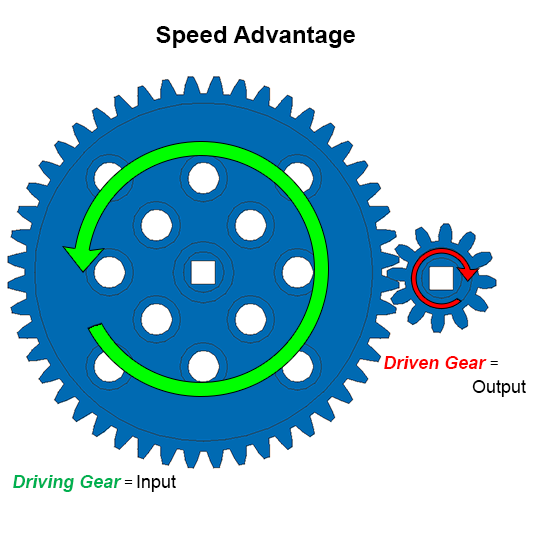Speed is a mechanical advantage that makes the output of the driven gear or machine faster. In this case, the M.A.D. Box has three times as much output as input rotations which makes it faster.

• Recorder, be sure to add notes to the engineering notebook about the mechanical advantage of speed within the M.A.D. Box.

### Step 3: M.A.D. Box's Compound Gear Ratios

• Build Expert, turn the handle connected to the 36 Tooth Gear slowly and let the group watch how fast the other handle turns.

• Recorder, after reading the description below, explain what a compound gear ratio is in the engineering notebook.

The gear ratio for the 36 Tooth Gear turning the 12 Tooth Gear was 1:3 with the mechanical advantage of speed. But when you turn the handle connected to the 36 Tooth Gear once, the other handle turns many more than three times.

That is because the M.A.D. Box uses a compound gear ratio. The M.A.D. Box's compound gear ratio is created by having 36 Tooth Gears and 12 Tooth Gears share the same shafts.

A compound gear ratio multiplies the mechanical advantage of speed or torque within a mechanism.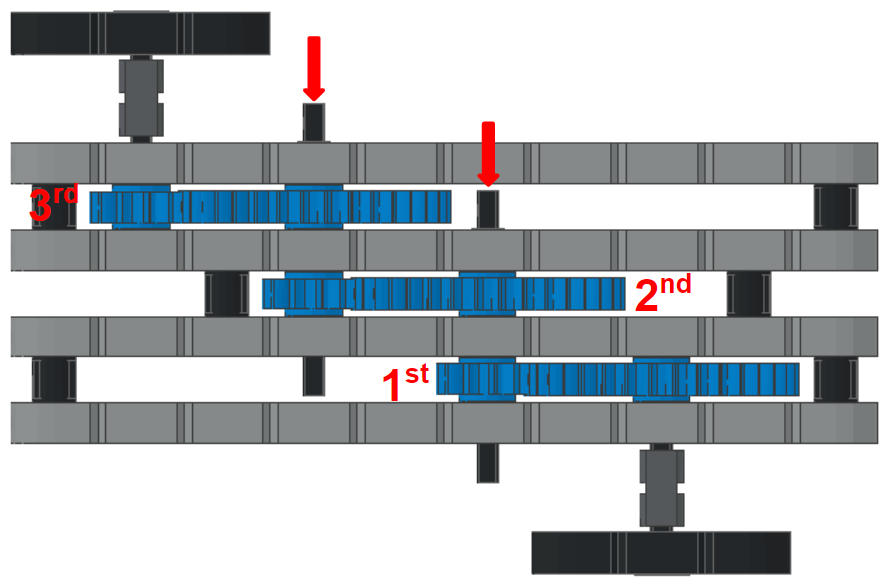Teacher Tips

The labels 1st, 2nd, and 3rd for the gear ratios in the image of the M.A.D. Box above are based on turning the handle connected to the 36 Tooth Gear. That is the handle at the bottom of the image.

The red arrows in the image above show the shafts that have both 36 Tooth and 12 Tooth Gears on them. Those shafts connect the first, second, and third gear ratios to each other. When the shaft turns, both the 12 Tooth and 36 Tooth Gears on the shaft turn.

This multiplies the mechanical advantage created by each gear ratio because they are connected into a compound gear ratio.

The M.A.D. Box has two compound gear ratios because you can give it input on either side - one leading to a torque advantage and the other leading to a speed advantage.

To calculate the compound gear ratio on one side of the M.A.D. Box, we need to find the three gear ratios in the build from that input to the output, and then multiply them by each other.

• Build Expert, find the side of the M.A.D. Box where the input handle turns the 36 Tooth Gear and show it to the group. Hint: It is the handle at the bottom of the image above. Point out in the build to review where the three gear ratios are found.

• Remember, all of the driving gears are 36 Tooth Gears and all of the driven gears are 12 Tooth Gears.

• Calculator and Recorder, complete and check the equations below: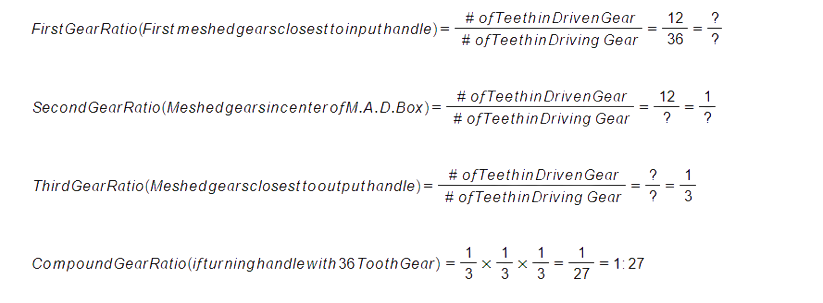• The entire team should try to answer the following questions: What does the 1:27 Compound Gear Ratio mean? When the handle with the 36 Tooth Gear is turned once, how many turns of the other handle should there be?

• The Recorder should organize the team's best answers and write them in the engineering notebook.Teacher Toolbox - Solution

The Calculator and Recorder in the group should recognize that the calculation should be completed as follows:

• The missing fraction in the First Gear Ratio is 1/3.

• The missing fractions in the Second Gear Ratio are 12/36 and 1/3.

• The missing fraction in the Third Gear Ratio is 12/36.

The equation for the Compound Gear Ratio calculation was provided because it was students' first time seeing it completed.

The 1:27 ratio means that for each time the first 36 Tooth Gear completes one full rotation, the last 12 Tooth Gear (the one closest to the output handle) rotates 27 times. That indicates that there is a mechanical advantage of speed.

Highlight the mechanical advantage of speed for students by asking them to turn the handle with the 36 Tooth Gear at a reasonable pace and notice how fast the output handle turns. Mathematically, the output handle is turning 27 times as fast as the input handle!

### Step 4: The M.A.D. Box's Compound Gear Ratio for Torque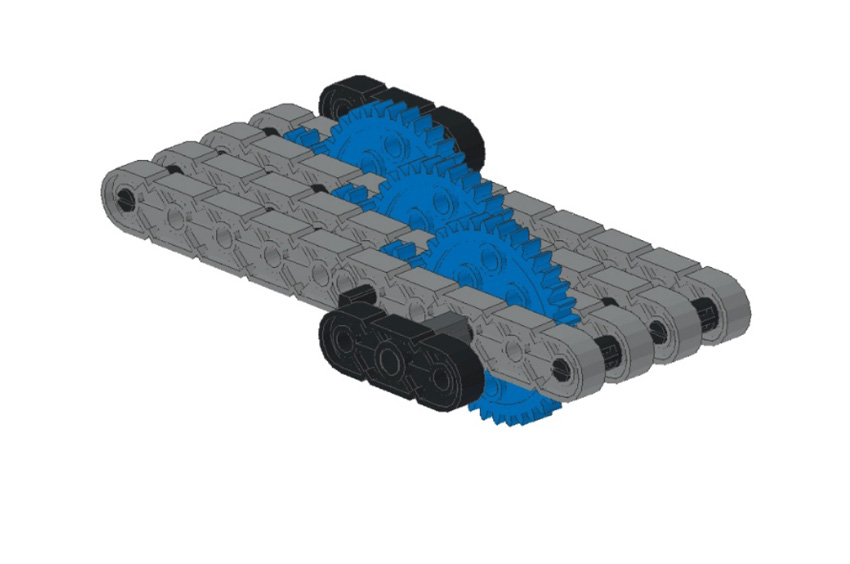• Build Expert, find the side of the M.A.D. Box where the input handle turns the 12 Tooth Gear and show it to the group. Hint: It is the opposite side of the M.A.D. Box as you were using above. Point out that when using this input handle, all of the driving gears are 12 Tooth Gears and all of the driven gears are 36 Tooth Gears.

• Calculator and Recorder, complete and check the equations below: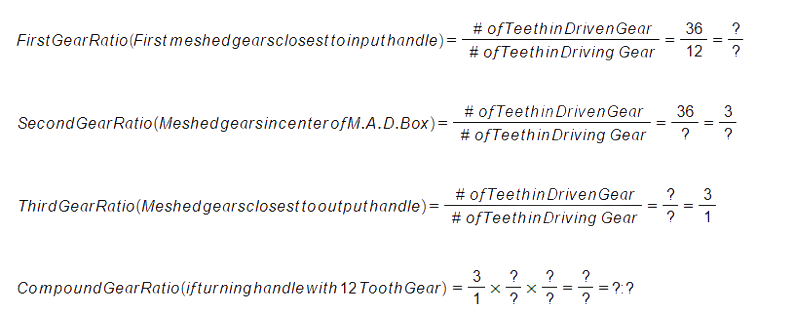• The entire team should try to answer the following questions: What is the Compound Gear Ratio and what does it mean? How many times do you turn the handle with the 12 Tooth Gear in order to turn the other handle once?

• The Recorder should organize the team's best answers and write them in the engineering notebook.Teacher Toolbox - Solution

The Calculator and Recorder in the group should recognize that the calculation should be completed as follows:

• The missing fraction in the First Gear Ratio is 3/1.

• The missing fractions in the Second Gear Ratio are 36/12 and 3/1.

• The missing fraction in the Third Gear Ratio is 36/12.

The equation for the Compound Gear Ratio calculation is missing 3/1 and 3/1 which yields 27/1 and the Compound Gear Ratio is 27:1.

The 27:1 ratio means that the first 12 Tooth Gear needs to complete 27 full rotations in order for the last 36 Tooth Gear (the one closest to the output handle) to rotate one full rotation. That indicates that there is a mechanical advantage of torque.

Highlight the mechanical advantage of torque for students by asking them to turn the handle with the 12 Tooth Gear at a reasonable pace and feel the force of the output handle as it turns. Mathematically, the output handle is turning with the force of 27 turns of the input handle!

### Step 5: Thinking about the M.A.D. Box's Design

Why aren't the M.A.D. Box's six gears all in one row?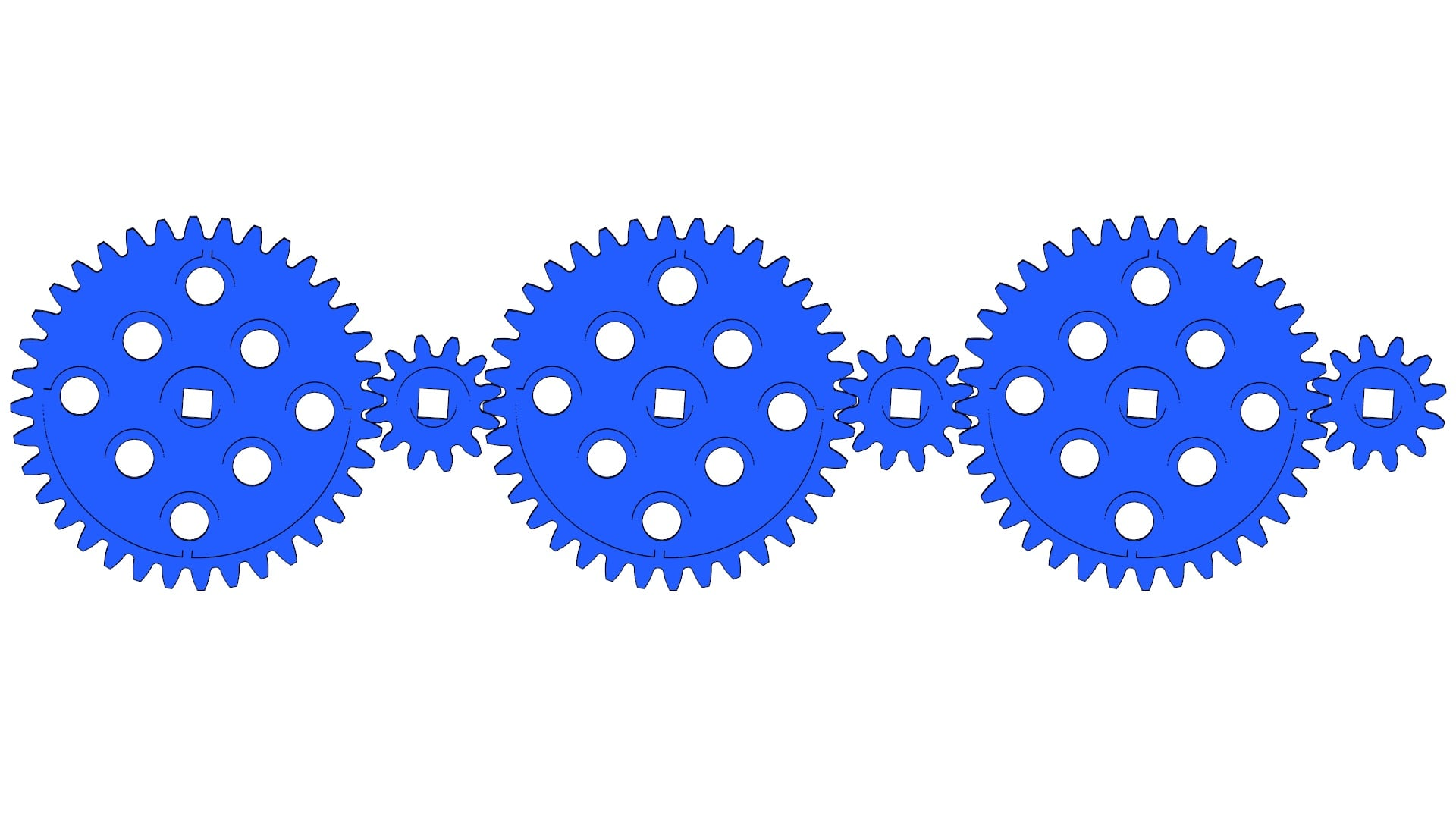A design where all of the gears are meshed in a line is called a gear train. The image above shows the M.A.D. Box's gears as a gear train.

A gear train like this only has one gear ratio and it is not a compound gear ratio. The ratio is either 1:3 or 3:1 depending on whether the first or last gear is the driving gear. Only the sizes of the first and last gears in this gear train matter to the gear ratio.

The gears between the first and last gears are called idler gears. They do not increase the power or speed. Idler gears only change the direction of the rotation.

Why wasn't the M.A.D. Box designed with only two gears: a small gear and a gear with 27 times more teeth?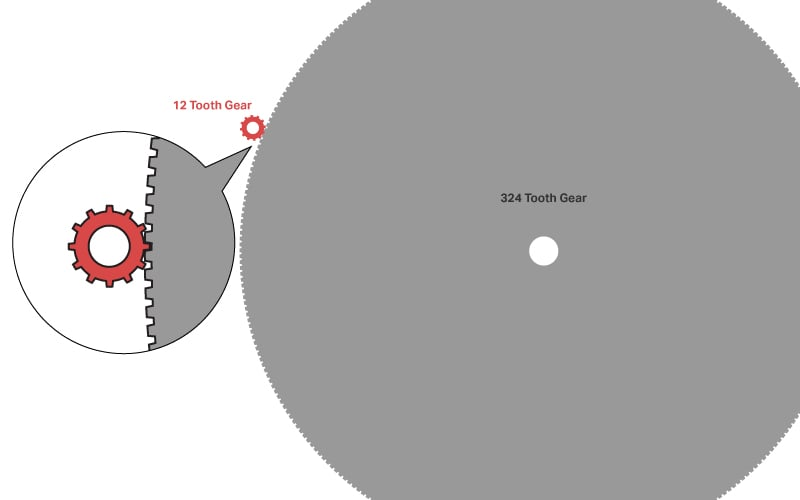The Compound Gear Ratio of the M.A.D. Box is 1:27 or 27:1. You might wonder why it wasn't designed with only two gears: the 12 Tooth Gear and a 324 Tooth Gear. That would have led to a 1:27 or 27:1 gear ratio.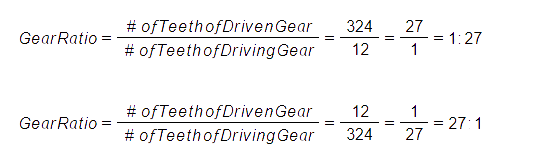There are two reasons why the M.A.D. Box wasn't designed with a 324 Tooth Gear.

The first reason is that a VEX Plastic 324 Tooth Gear doesn't exist. The largest gear in the kit is a 60 Tooth Gear. When engineers design builds, they need to take into account what materials are available and a 324 Tooth Gear was not available.

The second reason is that a 324 Tooth Gear, if available, would be very large. A gear that size would make the build difficult to handle. The compound gear ratio makes better sense for designing a handheld device. When engineers design builds, they need to take into account how the device will be used by consumers.Motivate Discussion - Summing Up

Students should finish this STEM Lab with an understanding of how gear ratios are important to the design of robots. In short, a given gear ratio is used either transfer power or to create torque or speed mechanical advantages for the robot's parts. Start with this discussion:

Q: To gain the mechanical advantage of speed, which is larger: the driving gear or the driven gear?
A: The driving gear is larger than the driven gear when creating speed.

Q: Why might you want to increase the speed of a robot?
A: The robot can then move and operate as quickly as possible.

Q: Why might you want to increase the torque on a robot? Hint: Consider its moving parts.
A: The robot might be heavy or need to move heavy objects. The increase in torque on its drivetrain (wheelbase) would help it to move. The increase in torque of its claw or arm can help to lift heavy objects.

Q: Why might you use a compound gear ratio instead of using two gears with a bigger difference in their numbers of teeth?
A: Some gear ratios are so large that a build could not have a gear(s) of that size. There might not even be a gear of the size needed to create that gear ratio. So compound gear ratios help engineers create greater mechanical advantages while minimizing the space required.

Q: Why might you include a gear train in a build? Does it increase torque or speed?
A: A gear train helps to transfer power but does not increase torque or speed any more than the ratio between the ending driving and driven gears on the train.

Students will return to thinking about this in the Apply section's page about Designing a Competition Robot for Torque or Speed. For further reading, there is a VEX Library article about How to Use Simple Gear Ratios. The article is written within the context of the VEX V5 robots but the concepts apply to VEX IQ robot designs as well.